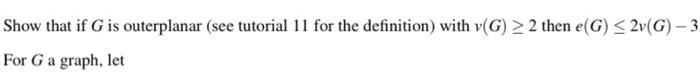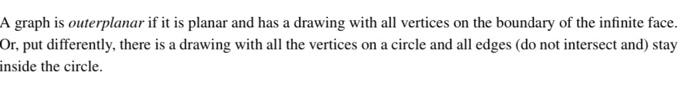Home / Expert Answers / Other Math / thats-all-i-have-show-that-if-g-is-outerplanar-see-tutorial-11-for-the-definition-with-v-pa525

# (Solved): thats all i have Show that if $$G$$ is outerplanar (see tutorial 11 for the definition) with $$v ...thats all i have Show that if \( G$$ is outerplanar (see tutorial 11 for the definition) with $$v(G) \geq 2$$ then $$e(G) \leq 2 v(G)-3$$ For $$G$$ a graph, let graph is outerplanar if it is planar and has a drawing with all vertices on the boundary of the infinite face. Dr, put differently, there is a drawing with all the vertices on a circle and all edges (do not intersect and) stay nside the circle.

We have an Answer from Expert